# Shear Force And Bending Moment - 3

## 10 Questions MCQ Test Topicwise Question Bank for GATE Mechanical Engineering | Shear Force And Bending Moment - 3

Description
This mock test of Shear Force And Bending Moment - 3 for Mechanical Engineering helps you for every Mechanical Engineering entrance exam. This contains 10 Multiple Choice Questions for Mechanical Engineering Shear Force And Bending Moment - 3 (mcq) to study with solutions a complete question bank. The solved questions answers in this Shear Force And Bending Moment - 3 quiz give you a good mix of easy questions and tough questions. Mechanical Engineering students definitely take this Shear Force And Bending Moment - 3 exercise for a better result in the exam. You can find other Shear Force And Bending Moment - 3 extra questions, long questions & short questions for Mechanical Engineering on EduRev as well by searching above.
QUESTION: 1

Solution:
QUESTION: 2

Solution:
QUESTION: 3

### The shear force diagram is shown below for a loaded beam. The corresponding bending moment diagram is represented by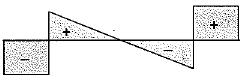Solution:
QUESTION: 4

A loaded beam PQRS is shown in the given figure.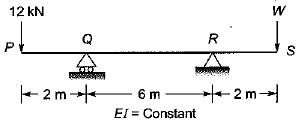The magnitude of reaction at R will be zero if the value of load W is

Solution:

For reaction at R to be zero, take moment about Q.
12 x 2 = W x 8
∴ W = 3 kN

QUESTION: 5

For a cantilever beam dM/dx = constant for its whole length. What is the shape of SF diagram for the beam?

Solution:
QUESTION: 6

For the beam loaded as shown in figure we use below. The magnitude of bending moment at the roller support is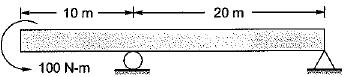Solution: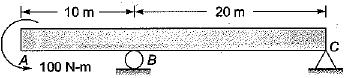Bending, moment at roller support
= Moment about B in Beam portion AB
= 100 N-m

QUESTION: 7

A concentrated load of P acts on a simply supported beam of span L at a distance L/3 from the left support. The bending moment at the point of application of the load is given by

Solution: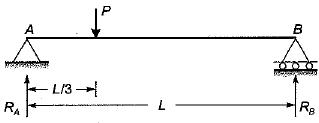Taking moment about A, we get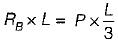∴ RB = P/3
and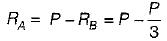RA = 2P/3
Bending moment at the point of application of load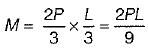QUESTION: 8

The bending moment diagram for a beam is a triangle with its base equal to length of the beam. What type of loading and beam does it represent?

Solution:
QUESTION: 9

List-I shows different loads acting on a beam and List-lI shows different bending moment distributions. Match List-I with List-ll and select the correct answer using the codes given below the lists:
List - I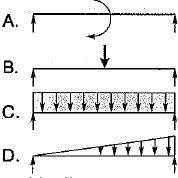List - II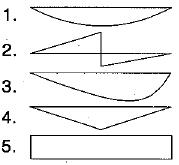Codes:
A B C D
(a)  4 2 1  3
(b)  5 4 1  3
(c)  2 5 3  1
(d)  2 4 1  3

Solution:
QUESTION: 10

Two identical cantilever beams are supported as shown, with their free ends in contact through a rigid roller. After the load Pis applied, the free ends will have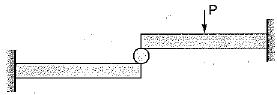Solution: